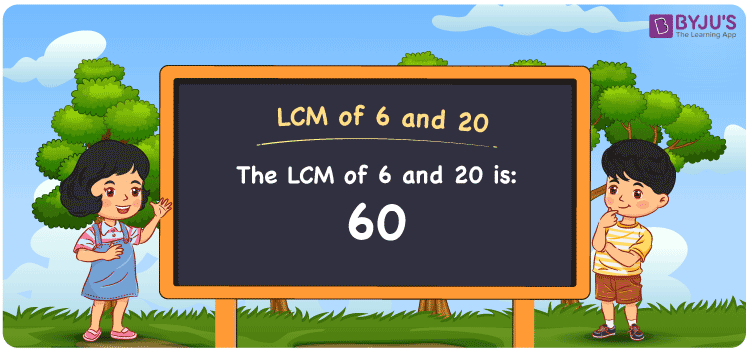Checkout JEE MAINS 2022 Question Paper Analysis : Checkout JEE MAINS 2022 Question Paper Analysis :

# LCM of 6 and 20

LCM of 6 and 20 is 60. The LCM value is the number evenly divisible by the given numbers. Least common multiple of 6 and 20 is the smallest number we obtain considering the common multiples. (6, 12, 18, 24, 30, ….) and (20, 40, 60, 80, 100,….) are the few multiples of 6 and 20. We can find the LCM easily with the help of various methods such as prime factorisation, division and by listing the multiples.

## What is LCM of 6 and 20?

The answer to this question is 60. The LCM of 6 and 20 using various methods is shown in this article for your reference. The LCM of two non-zero integers, 6 and 20, is the smallest positive integer 60 which is divisible by both 6 and 20 with no remainder.## How to Find LCM of 6 and 20?

LCM of 6 and 20 can be found using three methods:

• Prime Factorisation
• Division method
• Listing the multiples

### LCM of 6 and 20 Using Prime Factorisation Method

The prime factorisation of 6 and 20, respectively, is given by:

6 = 2 x 3 = 2¹ x 3¹

20 = 2 x 2 x 5 = 2² x 5¹

LCM (6, 20) = 60

### LCM of 6 and 20 Using Division Method

We’ll divide the numbers (6, 20) by their prime factors to get the LCM of 6 and 20 using the division method (preferably common). The LCM of 6 and 20 is calculated by multiplying these divisors.

 2 6 20 2 3 10 3 3 5 5 1 5 x 1 1

No further division can be done.

Hence, LCM (6, 20) = 60

### LCM of 6 and 20 Using Listing the Multiples

To calculate the LCM of 6 and 20 by listing out the common multiples, list the multiples as shown below

 Multiples of 6 Multiples of 20 6 20 12 40 18 60 24 80 30 100 36 120 42 140 48 160 54 180 60 200 66 220

The smallest common multiple of 6 and 20 is 60.

Therefore LCM (6, 20) = 60

## Video Lesson on Applications of LCM## LCM of 6 and 20 Solved Examples

Question: The LCM and GCD of two numbers are 60 and 2. If one number is 6, determine the other number.

Solution:

Consider m as the other number

GCD x LCM = 6 x m

m = (GCD x LCM)/6

m = (2 x 60)/6

m = 120/6

m = 20

Hence, the other number is 20.

## Frequently Asked Questions on LCM of 6 and 20

### How can the LCM of 6 and 20 be found?

The LCM of 6 and 20 is 60. We have to find the multiples of 6 and 20 to know the LCM and the smallest multiple that is divisible by 6 and 20 has to be determined.

### What are the methods used to get the LCM value of 6 and 20?

The methods used to get the LCM value of 6 and 20 are Prime Factorization Method, Division Method and Listing multiples.

### Calculate the GCF if the LCM of 6 and 20 is 60.

LCM x GCF = 6 x 20

Given

LCM of 6 and 20 = 60

60 x GCF = 120

GCF = 120/60 = 2